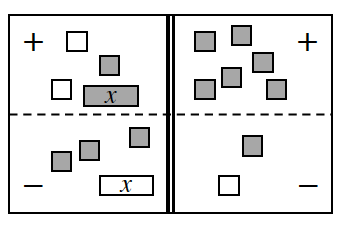### Home > CC3MN > Chapter 3 > Lesson 3.1.1 > Problem3-7

3-7.

On your paper, write the equation represented on the Equation Mat at right. Simplify as much as possible and then solve for $x$.Move all tiles in the "minus" region to the "plus" region by flipping the tiles and remove any zero pairs. Write the equation represented.

$2x-4=6$

Solve for $x$.

$2x−4=6$
$+4+4$

$\frac{2{\it x}}{2} = \frac{10}{2}$

$x=5$

Use the eTool below to help solve the problem.
Click the link at right for the full version of the eTool: 3-7 HW eTool (CPM)